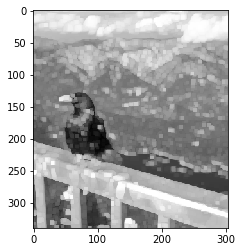In [ ]:
 
In :
%matplotlib inline
import tensorflow as tf
import matplotlib
from matplotlib import pyplot as plt
from skimage import io        # to read images
In :
# opening and viewing an image
image = io.imread("bird_pic_by_benjamin_planche.png") # 1 image uploaded 
In :
# 2 image viwing
print("Image shape: {}".format(image.shape))
plt.imshow(image, cmap=plt.cm.gray)

Image shape: (680, 608) 
Out:
<matplotlib.image.AxesImage at 0x2525f8f6ba8>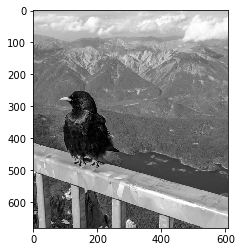In :
#converting the image into a tensor in order to do som tensorflow operations
image = tf.convert_to_tensor(image, tf.float32, name="input_image")
print("Tensor shape: {}".format(image.shape)) # testing image shape after tensor 
Tensor shape: (680, 608) 
In :
# we need to expand our image because tensorflow work on iamges of shape (B,W,H,D)
image = tf.expand_dims(image, axis=0) # we expand our tensor, adding a dimension at position 0
print("Tensor shape: {}".format(image.shape)) # testing the addition 
Tensor shape: (1, 680, 608) 
In :
image = tf.expand_dims(image, axis=-1) # we expand our tensor, adding a dimension at position 4
print("Tensor shape: {}".format(image.shape))# testing after the addtion 
Tensor shape: (1, 680, 608, 1) 

## Convolution

In :
#defining our kernal / filter Guassion Blur
kernel = tf.constant([[1 / 16, 2 / 16, 1 / 16],
[2 / 16, 4 / 16, 2 / 16],
[1 / 16, 2 / 16, 1 / 16]], tf.float32, name="gaussian_kernel")
In :
# reshaping the filter tensor to be of shape (k,k,N,D)
kernel = tf.expand_dims(tf.expand_dims(kernel, axis=-1), axis=-1)
In :
# atual filtering operation of the iamge
blurred_image = tf.nn.conv2d(image, kernel, strides=[1, 1, 1, 1], padding="SAME")
In :
blurred_res = blurred_image.numpy()
# We "unbatch" our result by selecting the first (and only) image; we also remove the depth dimension:
blurred_res = blurred_res[0, ..., 0]

plt.imshow(blurred_res, cmap=plt.cm.gray)

Out:
<matplotlib.image.AxesImage at 0x2525fea35f8>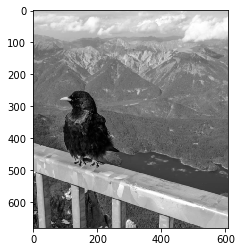In :
#defining a contour detection filter
kernel = tf.constant([[-1, -1, -1],
[-1,  8, -1],
[-1, -1, -1]], tf.float32, name="edge_kernel")
kernel = tf.expand_dims(tf.expand_dims(kernel, axis=-1), axis=-1)
In :
edge_image = tf.nn.conv2d(image, kernel, strides=[1, 2, 2, 1], padding="SAME")
edge_res = edge_image.numpy()[0, ..., 0]
plt.imshow(edge_res, cmap=plt.cm.gray)

Out:
<matplotlib.image.AxesImage at 0x252600c1e80>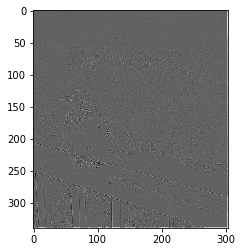looking closely to the iamge there is a white border countor that resulted from zero-padding padding "SAME", and it will disapear if we don't pad the image

In :
edge_image = tf.nn.conv2d(image, kernel, strides=[1, 2, 2, 1], padding="VALID")  # changing the padding to vaid
edge_res = edge_image.numpy()[0, ..., 0]
plt.imshow(edge_res, cmap=plt.cm.gray)

Out:
<matplotlib.image.AxesImage at 0x25260138c50>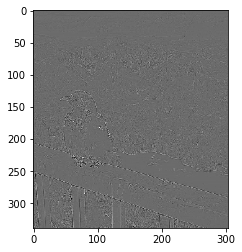## Pooling

In :
# averge pooling
avg_pooled_image = tf.nn.avg_pool(image, ksize=[1, 2, 2, 1], strides=[1, 2, 2, 1], padding="SAME")
avg_res = avg_pooled_image.numpy()[0, ..., 0]
plt.imshow(avg_res, cmap=plt.cm.gray)

Out:
<matplotlib.image.AxesImage at 0x2526019d828>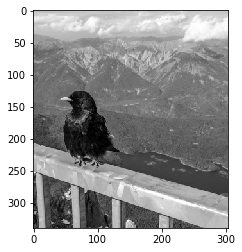In :
# max pooling
max_pooled_image = tf.nn.max_pool(image, ksize=[1, 10, 10, 1], strides=[1, 2, 2, 1], padding="SAME")
max_res = max_pooled_image.numpy()[0, ..., 0]
plt.imshow(max_res, cmap=plt.cm.gray)

Out:
<matplotlib.image.AxesImage at 0x25260214160>